Operations on Graph and Special Graphs using Networkx module | Python

• Last Updated : 19 Jul, 2019

Prerequisite : Create an undirected Graph.

The basic Graph operations are as follows:

Getting Subgraph from a Graph:

Given a Graph, and a subset of its set of nodes, we can create a Subgraph by selecting these nodes and all the edges between them as were present in the original Graph. The following code will clearly illustrate this operation.

 import networkx as nx import matplotlib.pyplot as plt  G = nx.Graph()  plt.figure(figsize =(9, 12))G.add_edges_from([(1, 2), (1, 3), (2, 3), (2, 4), (2, 5), (3, 4),                          (4, 5), (4, 6), (5, 7), (5, 8), (7, 8)])  # original Graph createdplt.subplot(211)print("The original Graph:")  nx.draw_networkx(G)

The original Graph: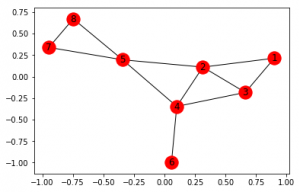H = G.subgraph([1, 2, 3, 4])# [1, 2, 3, 4] is the subset of # the original set of nodes  plt.subplot(212)print("The Subgraph:")nx.draw_networkx(H)

The Subgraph: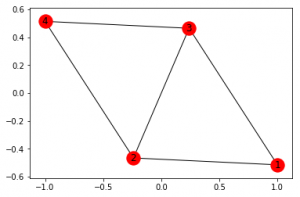The original Graph G has nodes from 1 to 8. We have selected nodes 1, 2, 3 and 4 and created a Subgraph H which has 5 edges which were present among them in the original graph G.

Union of two Graphs:

Given two graphs G and H, the union of the 2 graphs create a single Graph which may have multiple connected components. But we have to keep in mind that the set of nodes of G and H should be disjoint, in other words, the two graphs shouldn’t have any nodes in common.

 import networkx as nx import matplotlib.pyplot as plt  G = nx.Graph()  plt.figure(figsize =(9, 12))G.add_edges_from([(1, 2), (1, 3), (2, 3), (2, 4), (2, 5), (3, 4),                          (4, 5), (4, 6), (5, 7), (5, 8), (7, 8)])  # First Graph createdplt.subplot(311)nx.draw_networkx(G)  H = nx.Graph()H.add_edges_from([(13, 14), (13, 15), (13, 9),                  (14, 15), (15, 10), (9, 10)])  # Second Graph createdplt.subplot(312)nx.draw_networkx(H)    I = nx.union(G, H)plt.subplot(313)nx.draw_networkx(I)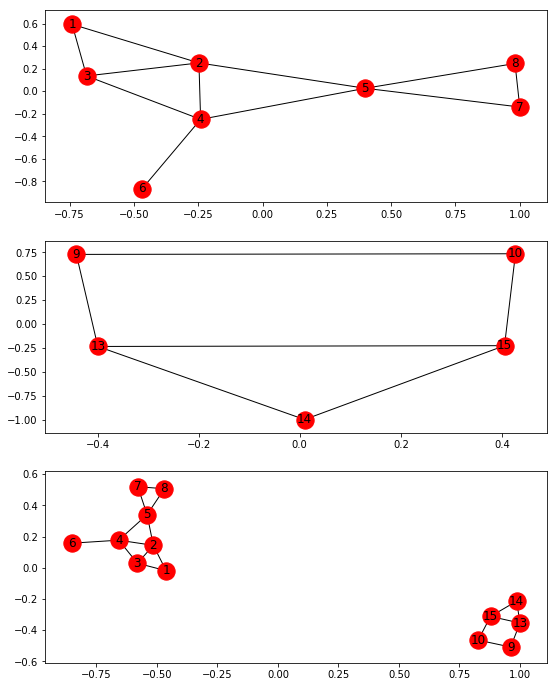The newly formed graph I is the union of graphs g and H. If we do have common nodes between two graphs and still want to get their union then we will use another function called disjoint_set()

I = nx.disjoint_set(G, H)

This will rename the common nodes and form a similar Graph.

Cartesian Product of two Graphs:

Given two graphs, G and H the cartesian product creates a new Graph, I = G*H. The set of nodes of I is the cartesian product of sets of nodes of G and H that is, V(I) = V(G)*V(H).

An edge ((), ()) exists if and only if:

• i=k andexists as an edge in H
• j=l andexists as an edge in G
 import networkx as nx import matplotlib.pyplot as plt  G = nx.Graph()  plt.figure(figsize =(9, 18))G.add_edges_from([(1, 2), (2, 3)])  # First Graph createdplt.subplot(311)nx.draw_networkx(G)  H = nx.Graph()H.add_edges_from([(6, 7)])# Second Graph createdplt.subplot(312)nx.draw_networkx(H)    I = nx.cartesian_product(G, H)plt.subplot(313)nx.draw_networkx(I)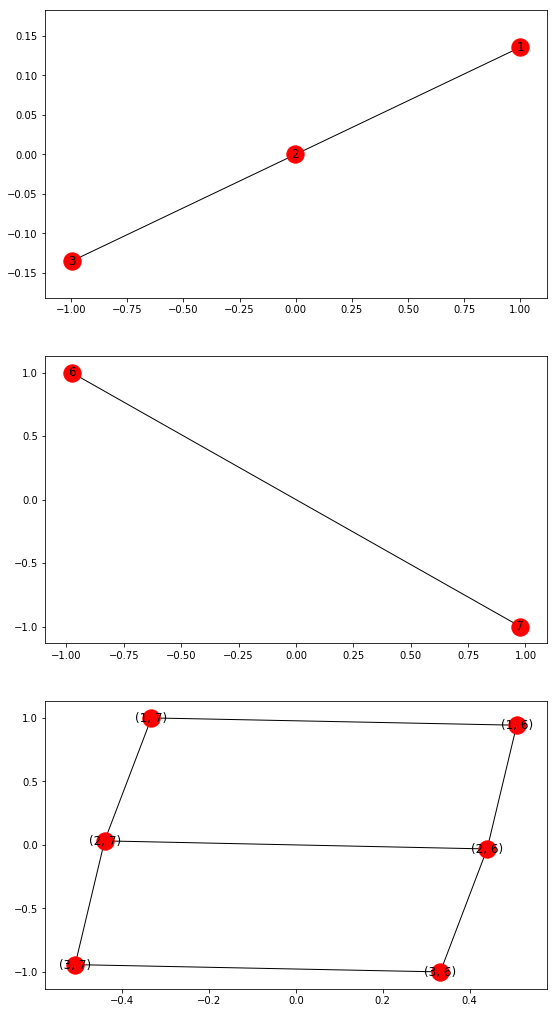This representation clearly shows how the product of the first 2 graphs result in the third graph.

Composition of two graphs:

Given two graphs G and H, if they have no common nodes then the composition of the two of them will result in a single Graph with 2 connected components (assuming G and H are connected graphs). This is the same result that we will obtain if we use nx.union(G, H) or nx.disjoint_union(G, H).
But if G and H have common nodes the resultant composition of these two graphs will result in a single connected Graph in such a way that G and H are subgraphs of the new Graph.

 import networkx as nx import matplotlib.pyplot as plt  G = nx.Graph()  plt.figure(figsize =(9, 15))G.add_edges_from([(1, 2), (1, 3), (2, 3), (2, 4)])  # First Graph createdplt.subplot(311)nx.draw_networkx(G)  H = nx.Graph()H.add_edges_from([(3, 7), (7, 4), (3, 4)])# Second Graph createdplt.subplot(312)nx.draw_networkx(H)    I = nx.compose(G, H)plt.subplot(313)nx.draw_networkx(I)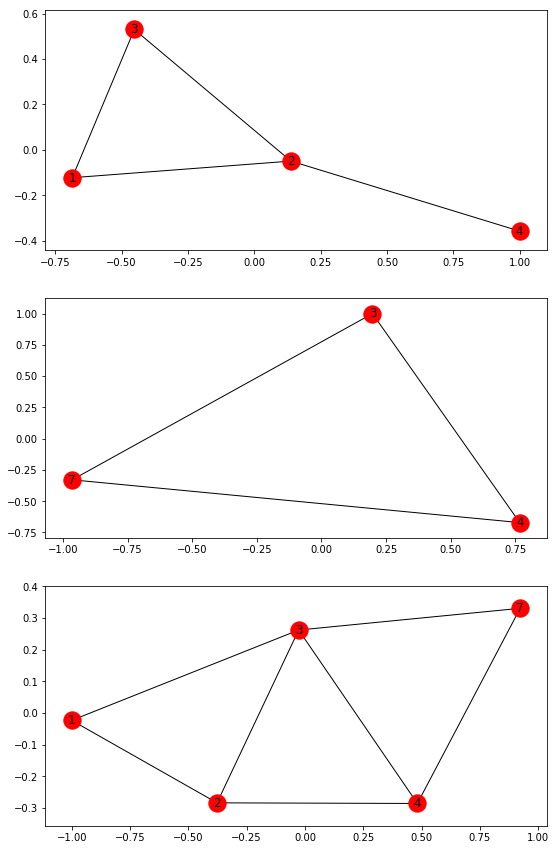The diagrams clearly visualize how the first two graphs compose together to form the third Graph.

Complement of a Graph:

Given a graph G, the complement of G (say, H) has all the nodes of G. It has all the possible edges that G does not have. Let V and E be the set of nodes and edges of G, then H has {(|V|*(|V|-1))/2 - |E|} number of edges. Thus the complement of a complete Graph will have no edges.

 import networkx as nx import matplotlib.pyplot as plt  G = nx.Graph()  plt.figure(figsize =(9, 16))G.add_edges_from([(1, 2), (1, 3), (2, 3), (2, 4)])  # Original Graph createdplt.subplot(211)nx.draw_networkx(G)  H = nx.complement(G)plt.subplot(212)nx.draw_networkx(H)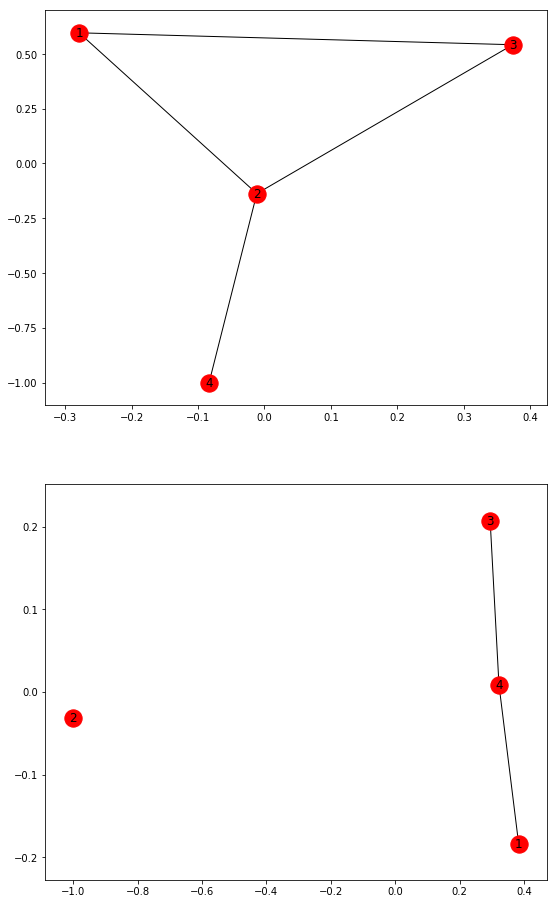Convert to Directed:

Given an undirected Graph G, this Networkx function will convert it to a Directed Graph by replacing its edges with 2-way Directed edges.

 import networkx as nx import matplotlib.pyplot as plt  G = nx.Graph()  plt.figure(figsize =(9, 16))G.add_edges_from([(1, 2), (1, 3), (2, 3), (2, 4)])# Original Undirected Graph created  plt.subplot(211)nx.draw_networkx(G)  H = nx.to_directed(G)plt.subplot(212)nx.draw_networkx(H)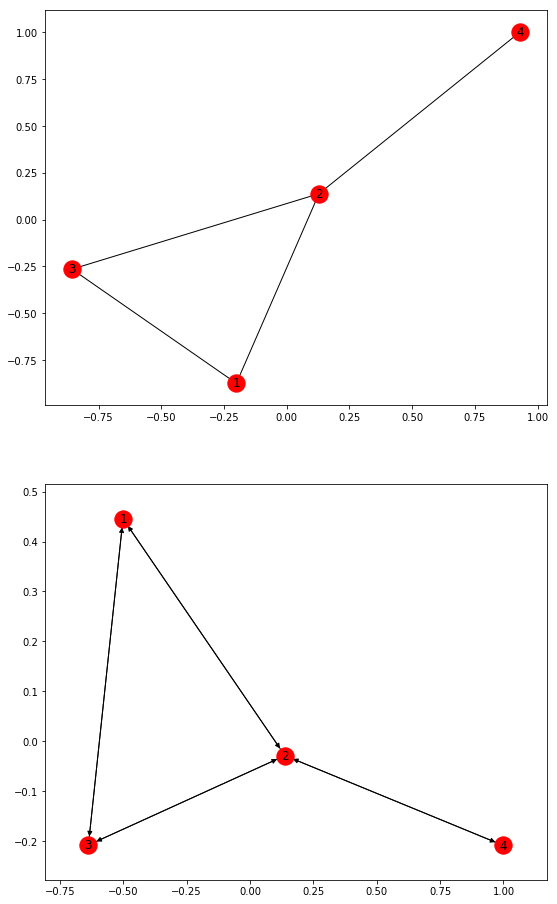Convert to Undirected:

Given a Directed Graph G, this Networkx function will convert it to an Undirected graph by converting all its directed edges to undirected edges. If two edges exist between a pair of nodes with different attributes (weights, colour etc.), then only one edge is created with an arbitrary choice of which edge data to use.

 import networkx as nx import matplotlib.pyplot as plt  G = nx.DiGraph()  plt.figure(figsize =(9, 16))G.add_edges_from([(1, 2), (1, 3), (2, 4)])  # Original Directed Graph createdplt.subplot(211)nx.draw_networkx(G)  H = nx.to_undirected(G)plt.subplot(212)nx.draw_networkx(H)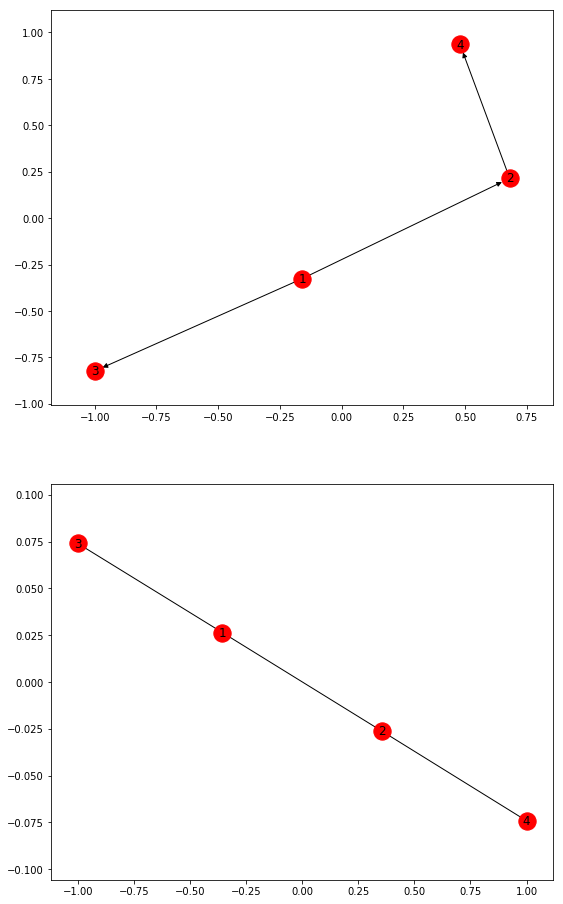Now, we will discuss the various Special Graphs offered by Networkx module.

Petersen Graph: The Petersen graph is an undirected graph with 10 vertices and 15 edges. It is a small graph that serves as a useful example and counterexample for many problems in graph theory.

 import networkx as nx import matplotlib.pyplot as plt  plt.figure(figsize =(9, 100))  # Peterson Graphplt.subplot(12, 1, 1)G = nx.petersen_graph()nx.draw_networkx(G)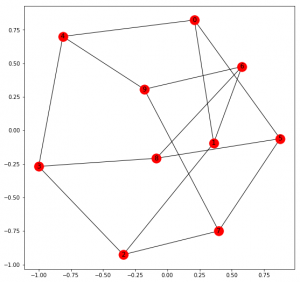Tutte Graph: The Tutte graph is a 3-regular graph with 46 vertices and 69 edges. The Tutte graph is a cubic polyhedral graph, but is non-hamiltonian. Therefore, it is a counterexample to Tait’s conjecture. that every 3-regular polyhedron has a Hamiltonian cycle.

 # Tutte Graphplt.subplot(12, 1, 2)G = nx.tutte_graph()nx.draw_networkx(G)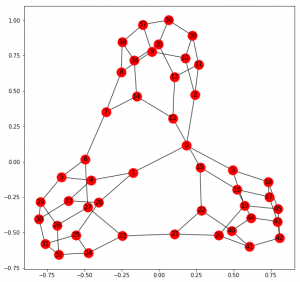Sedgewick Maze Graph: Sedgewick’s Maze Algorithm is used to generate large mazes. Networkx function pertaining to this method returns a small maze with a cycle.

 # Sedgewick Maze Graphplt.subplot(12, 1, 3)G = nx.sedgewick_maze_graph()nx.draw_networkx(G)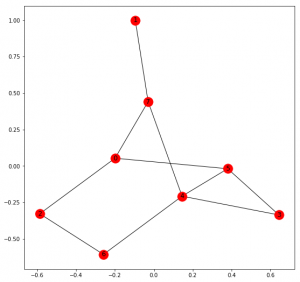Tetrahedral Graph: This returns a complete Graph with four nodes which are in the shape of a tetrahedron.

 # Tetrahedral Graphplt.subplot(12, 1, 4)G = nx.tetrahedral_graph()nx.draw_networkx(G)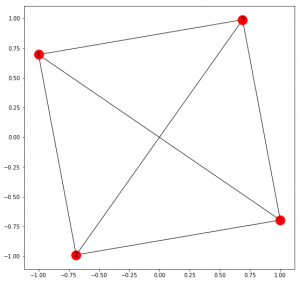Complete Graph: Returns a Complete Graph with given number of edges.

 # Complete Graph with 5 nodesplt.subplot(12, 1, 5)G = nx.complete_graph(6)nx.draw_networkx(G)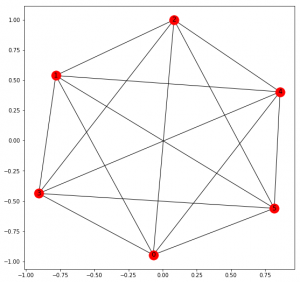Complete Bipartite Graph: Given two numbers n and m, it returns a Graph with two sets of n and m nodes in such a way that a node of one set is connected to all the nodes of the other set but with no node of its own set. This type of Graph is known as Bipartite.

 # Complete Bipartite Graph with 5 and 3 nodesplt.subplot(12, 1, 6)G = nx.complete_bipartite_graph(5, 3)nx.draw_networkx(G)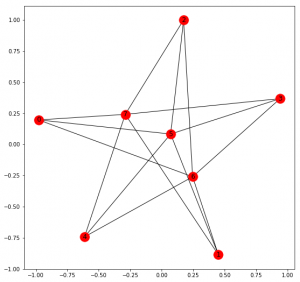Barbell Graph: Given two parameters n and m, it returns a Graph with two cliques of n nodes which are connected via m nodes in between.

 # Barbell Graph with clique of 4 and bridging of 2 nodesplt.subplot(12, 1, 7)  G = nx.barbell_graph(4, 2)nx.draw_networkx(G)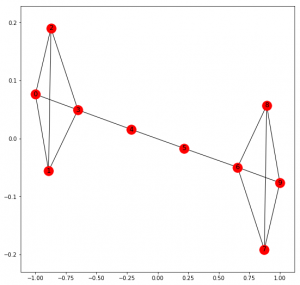Lollipop Graph: Given two parameters n and m, it returns a Graph with a clique of n vertices connected to a path of m nodes.

 # Lollipop Graph with clique of 5 and path of 2 nodesplt.subplot(12, 1, 8)G = nx.lollipop_graph(5, 2)nx.draw_networkx(G)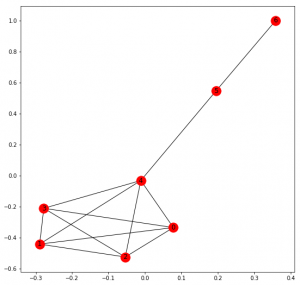Erdos Renyi Graph: Given two parameters n and p, it returns a Graph with n nodes with a probability of p for selecting each edge. For further details, see this article.

 # Erdos Renyi Graph with 20 nodes and probability of 0.25plt.subplot(12, 1, 9)  G = nx.erdos_renyi_graph(20, 0.25)nx.draw_networkx(G)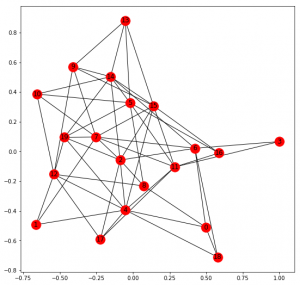Watts Strogatz Graph: Given three parameters n, k and p, returns a Small World Network of n nodes each of which is connected to k nearest neighbors with a probability of p of rewiring each edge.

 # Watts Strogatz Graph with 20 nodes, # 4 neighbours and probability of 0.2plt.subplot(12, 1, 10)G = nx.watts_strogatz_graph(20, 4, 0.2)nx.draw_networkx(G)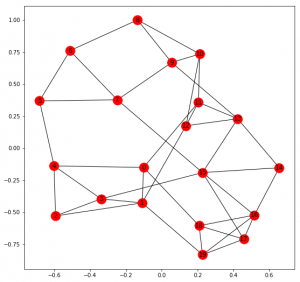Barabasi Albert Graph: Given two parameters n and m, returns a Barabasi Albert preferential attachment graph with n nodes and m number of edges to attach from a new node to existing nodes.

 # Barabasi Albert Graph with 20 nodes and 3 attaching nodesplt.subplot(12, 1, 11)G = nx.barabasi_albert_graph(20, 3)nx.draw_networkx(G)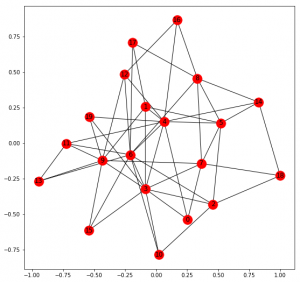Random Lobster Graph: Given three parameters n, p1 and p2, returns a lobster Graph. A lobster is a tree that reduces to a caterpillar when pruning all leaf nodes. A caterpillar is a tree that reduces to a path graph when pruning all leaf nodes. Graph has n nodes in backbone, p1 probability of adding an edge to the backbone, p2 probability of adding an edge a level beyond backbone.

 # Random Lobster Graph with 30 base# nodes and probabilites of 0.8plt.subplot(12, 1, 12)  G = nx.random_lobster(30, 0.8, 0.8)nx.draw_networkx(G)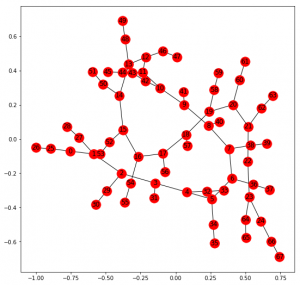My Personal Notes arrow_drop_up